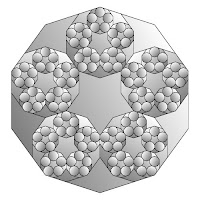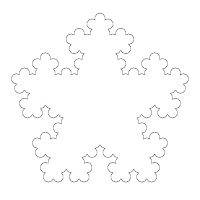## Thursday, December 9, 2010

### Girih tiling based on a decagonal fractal

My last entry described a girih tiling based on a pentagonal fractal. It's also possible to base a fractal tiling on decagons. This process is simply nesting or subdividing a decagon with five smaller decagons. Starting with a regular decagon, place five smaller regular decagons, edge-to-edge, within the original decagon. One vertex each of the smaller decagons should coincide with a vertex of the larger. Two sides each of the smaller decagons should be edge-to-edge with another small decagon. The lengths of the edges of the smaller decagon are 1/(2x(1+cosine(72))) of the larger. This is the same as calculating the sides of the five smaller decagons by dividing the larger decagon sides by the golden ratio, twice. That is, divide the length of a side of the original decagon by approximately 1.618, then divide the result again by approximately 1.618. The result is a measure for the side of a smaller decagon to nest and repeat inside a larger. The process of subdividing or nesting decagons with five smaller decagons may be repeated infinitely. Following are diagrams of nesting decagons, and a fractal border.Here's a completed girih tiling.